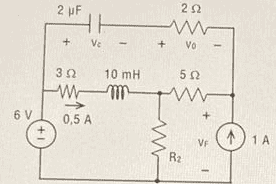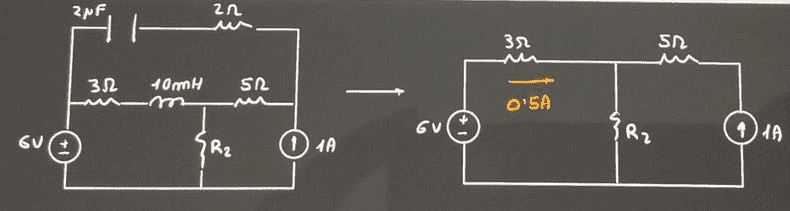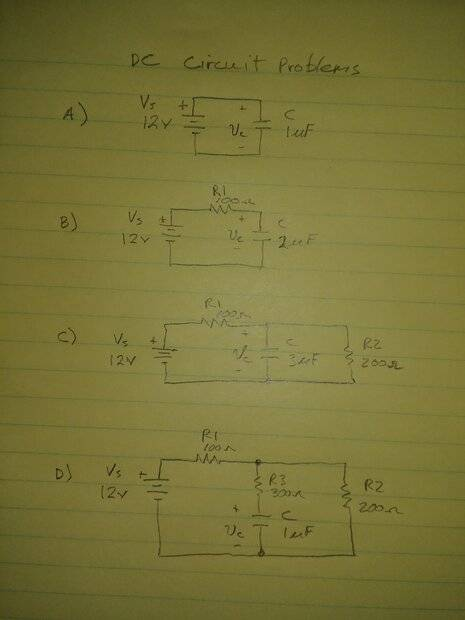# Capacitor circuit analysis

• Guillem_dlc
No. You can have a constant (DC) voltage across a capacitor. You can have a constant (DC) current through an inductor. Redraw your schematic with the changes you proposed and solve it, don't assume the solution.But it will be wrong, won't it?I don't understand. What will be wrong?It will be wrong if you use the assumption that the voltage across the capacitor is always 0.

#### Guillem_dlc

Homework Statement
In the circuit of the figure, determine:
a) ##R_2##, ##v_c##, ##v_0## and ##v_F##.
b) ##R'## to be connected in series with ##R_2## so that the voltage source neither yields nor absorbs power.
Relevant Equations
Capacitor: open circuit.
Figure:My attempt at a solution:Once the capacitors have been applied, we see directly that ##\boxed{v_c=v_0=0\, \textrm{V}}##
Wouldn't this one be done like this?
I know the following: in a circuit with capacitors and coils, if we have
• Capacitor: we change for an open circuit.
• Coil: we change for a short circuit.
I don't know if the capacitor didn't act as an open circuit while charging and once charged as a short circuit?

I assume those are DC sources, since otherwise there's information missing. So,
Guillem_dlc said:
I know the following: in a circuit with capacitors and coils, if we have
• Capacitor: we change for an open circuit.
• Coil: we change for a short circuit.
Yes, for DC circuits. DC current can't flow through a capacitor, and DC voltage can't stay across an inductor.

edit: OTOH, DC voltage can be across a capacitor and DC current can flow through an inductor. The resistance of a ideal capacitor is infinite. The resistance of an ideal inductor is zero.

Guillem_dlc said:
I don't know if the capacitor didn't act as an open circuit while charging and once charged as a short circuit?
That's why it's important to distinguish between DC circuit analysis and other situations (transients or AC steady state). The charging behavior just isn't counted for DC analysis, that is a transient solution. Capacitors and inductors behave differently in those other situations. Ask, if you want to know more about that. However, you will learn it in time as your studies continue. It's not part of this question, IMO.

Guillem_dlc said:
Once the capacitors have been applied, we see directly that vc=v0=0V

DaveE said:
Because with these notes we were left with an open circuit:
in a circuit with capacitors and coils, if we have
• Capacitor: we change for an open circuit.
• Coil: we change for a short circuit.
So there is no intensity, right?

Guillem_dlc said:
Because with these notes we were left with an open circuit:
in a circuit with capacitors and coils, if we have
• Capacitor: we change for an open circuit.
• Coil: we change for a short circuit.
Yes.

Guillem_dlc said:
So there is no intensity, right?
No. You can have a constant (DC) voltage across a capacitor. You can have a constant (DC) current through an inductor. Redraw your schematic with the changes you proposed and solve it, don't assume the solution.

But it will be wrong, won't it?

Guillem_dlc said:
But it will be wrong, won't it?
I don't understand. What will be wrong?

Try each of these DC analysis problems. In each case solve for the capacitor voltage. Let me know if any confuse you and why, if possible.DaveE said:
I don't understand. What will be wrong?
Because ##v_c## will be ##0## if I do as I have done, right?

OK in my problem A), what is the voltage across the capacitor if you remove the capacitor by replacing it with an open circuit (i.e. no connection).

DaveE said:
OK in my problem A), what is the voltage across the capacitor if you remove the capacitor by replacing it with an open circuit (i.e. no connection).
But how is this voltage calculated?

In the first ##v_c## it would be ##12##, wouldn't it?

In the second it would be ##12-V_{\textrm{resistance}}##

The c would be ##v_c=0##, wouldn't it?

And d I would say it would be ##12-V_{\textrm{res 100}}-V_{\textrm{res 300}}##

I would say it's like that, but I have no idea...

Guillem_dlc said:
The c would be ##v_c=0##, wouldn't it?
OK not in the c would be ##12-V_{\textrm{res 100}}##

Guillem_dlc said:
Homework Statement:: In the circuit of the figure, determine:
a) ##R_2##, ##v_c##, ##v_0## and ##v_F##.
b) ##R'## to be connected in series with ##R_2## so that the voltage source neither yields nor absorbs power.
Relevant Equations:: Capacitor: open circuit.

Once the capacitors have been applied, we see directly that vc=v0=0V
Wouldn't this one be done like this?
Vc = Vo = 0, means that if you put a voltmeter across the terminals of the capacitor, or across the terminals of the resistor, the voltmeter will register 0v. Which of course subsequently means that both terminals are at the same potential.
If that is the case( which it may or may not be - to be determined ), the solution is quite easy.

256bits said:
Vc = Vo = 0, means that if you put a voltmeter across the terminals of the capacitor, or across the terminals of the resistor, the voltmeter will register 0v. Which of course subsequently means that both terminals are at the same potential.
If that is the case( which it may or may not be - to be determined ), the solution is quite easy.
But it is not 0 is it?

From what I've seen, a capacitor always has a potential drop if it's charging, right?

Guillem_dlc said:
But how is this voltage calculated?
It is given to you by the voltage source (battery). You don't calculate it. A voltage source, by definition, will do whatever it takes, by sourcing or sinking current, to make the voltage at its terminals equal to the definition given; 12V in this case.

Guillem_dlc said:
In the first vc it would be 12, wouldn't it?
Yes. See above comment.

Guillem_dlc said:
In the second it would be 12−Vresistance
Yes. So what is Vresistance? What information do you need to find the voltage across a resistor?

Guillem_dlc said:
The c would be vc=0, wouldn't it?
No.
Guillem_dlc said:
And d I would say it would be 12−Vres 100−Vres 300
Yes. But now you'll have to understand how to find the value of those voltages across the resistors.

I think the original problem is a bit too complex for you at this stage. You need to become more familiar with Ohm's Law, Kirchhoff's Voltage Law, and Kirchhoff's Current Law to solve these problems. Khan Academy has some really good tutorials on this material. You should study those, I think.

DaveE said:
It is given to you by the voltage source (battery). You don't calculate it. A voltage source, by definition, will do whatever it takes, by sourcing or sinking current, to make the voltage at its terminals equal to the definition given; 12V in this case.

Yes. See above comment.

Yes. So what is Vresistance? What information do you need to find the voltage across a resistor?

No.

Yes. But now you'll have to understand how to find the value of those voltages across the resistors.

I think the original problem is a bit too complex for you at this stage. You need to become more familiar with Ohm's Law, Kirchhoff's Voltage Law, and Kirchhoff's Current Law to solve these problems. Khan Academy has some really good tutorials on this material. You should study those, I think.
Yes, I understand it already, thank you.